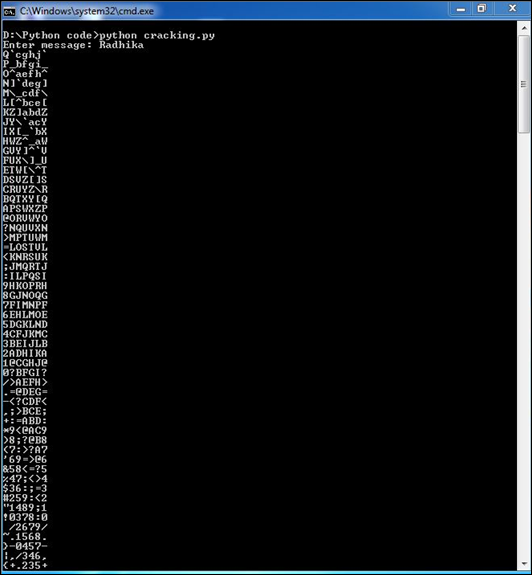# Python Forensics - Cracking an Encryption

In this chapter, we will learn about cracking a text data fetched during analysis and evidence.

A plain text in cryptography is some normal readable text, such as a message. A cipher text, on the other hand, is the output of an encryption algorithm fetched after you enter plain text.

Simple algorithm of how we turn a plain text message into a cipher text is the Caesar cipher, invented by Julius Caesar to keep the plain text secret from his enemies. This cipher involves shifting every letter in the message "forward" by three places in the alphabet.

Following is a demo illustration.

a → D

b → E

c → F

....

w → Z

x → A

y → B

z → C

## Example

A message entered when you run a Python script gives all the possibilities of characters, which is used for pattern evidence.

The types of pattern evidences used are as follows −

• Tire Tracks and Marks
• Impressions
• Fingerprints

Every biometric data comprises of vector data, which we need to crack to gather full-proof evidence.

The following Python code shows how you can produce a cipher text from plain text −

```import sys

def decrypt(k,cipher):
plaintext = ''

for each in cipher:
p = (ord(each)-k) % 126

if p < 32:
p+=95
plaintext += chr(p)
print plaintext

def main(argv):
if (len(sys.argv) != 1):
sys.exit('Usage: cracking.py')
cipher = raw_input('Enter message: ')

for i in range(1,95,1):
decrypt(i,cipher)

if __name__ == "__main__":
main(sys.argv[1:])
```

## Output

Now, check the output of this code. When we enter a simple text "Radhika", the program will produce the following cipher text.